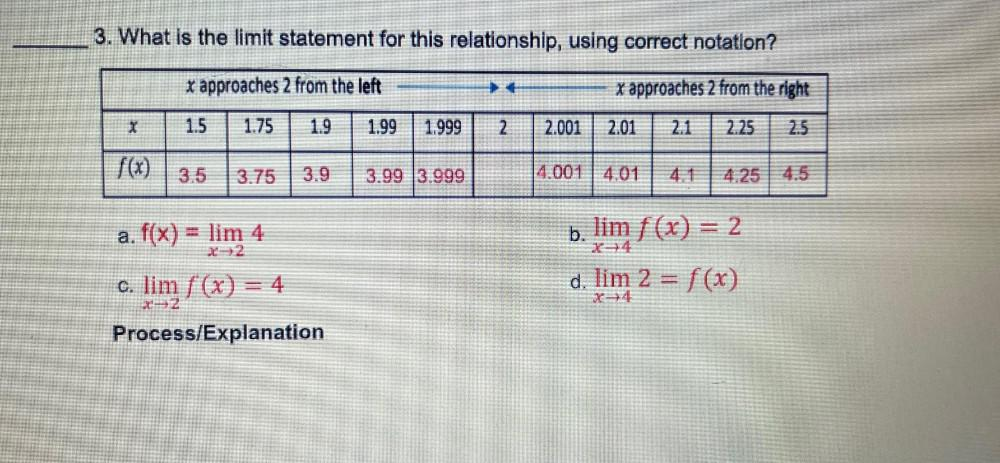Question:

# What is the limit statement for this relationship, using correct notation? (x approaches 2 from the left) ---> <---(x approaches 2 from the right) x 1.5 1.75 1.9 1.99 1.999 2 2.What is the limit statement for this relationship, using correct notation? (x approaches 2 from the left) ---> <---(x approaches 2 from the right) x 1.5 1.75 1.9 1.99 1.999 2 2.001 2.01 2.1 2.25 2.5 f(x) 3.5 3.75 3.9 3.99 3.999 4.001 4.01 4.1 4.25 4.5 a. f(x) = lim 4 x->2 b. lim f(x) = 2 x->4 c. lim f(x) = 4 x->2 d. lim 2 = f(x) x->4 Process/Explanation